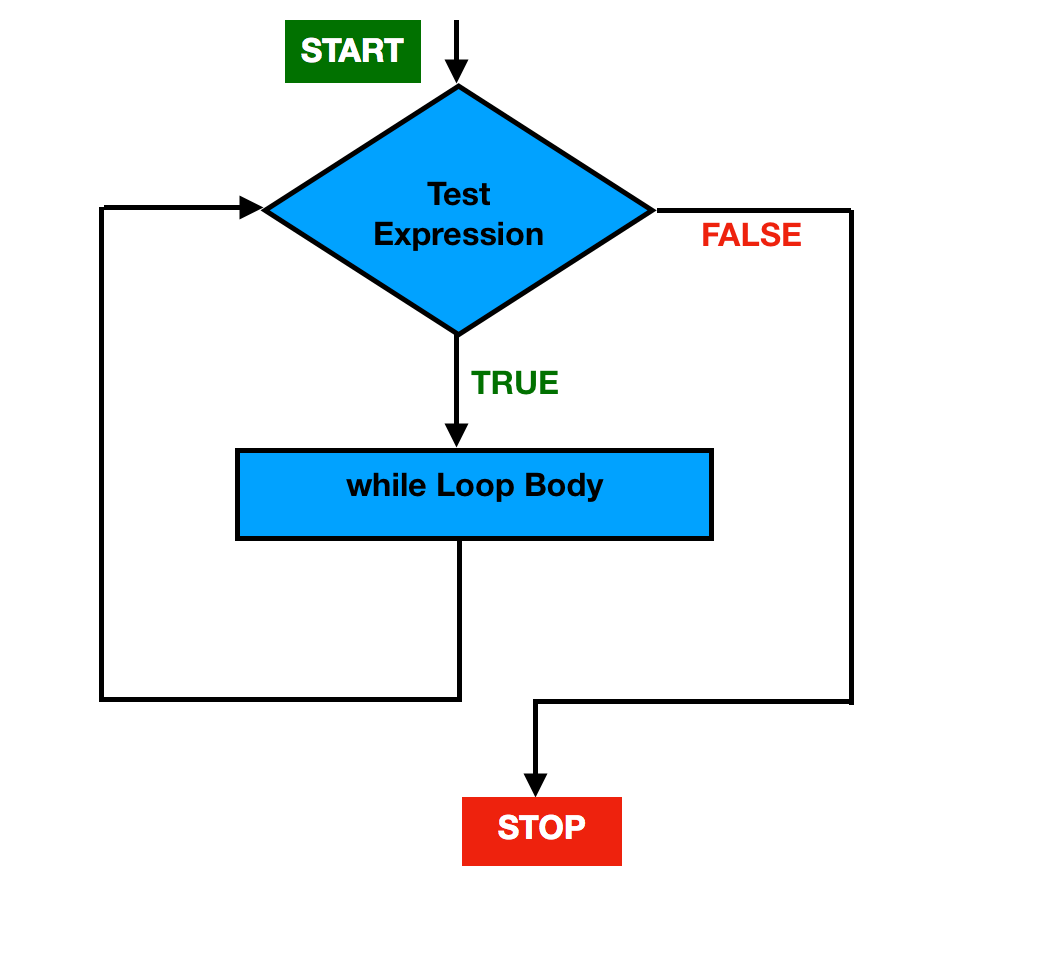# Java while Loop

In this article, you will learn about while loop and how to create while loop in Java programming.

Loops in Java are used when we want to execute a block of statements repeatedly until a specific condition is met(condition is false).

### Java While Loop

The while loop executes the block of code as long as a specified condition is true.

#### Syntax

```//while loop

//test_expression is a boolean expression
while (test_expression) {

//body of the loop
//statements to be executed
}```

### How while loop works?

When the Control falls into the while loop, it first checks the test_expression (boolean expression).

If the test expression is evaluated to true,

• The statements inside the body of the loop get executed.
• Then, the test expression is evaluated again and this process goes on until the test expression is evaluated to false.

If the test expression is evaluated to false,

• while loop gets terminated and control goes out of the while loop.

#### Flowchart#### Example 1

```public class WhileLoop {

public static void main(String[] args) {

//initialization
int i = 1;

//exit when i becomes greater than 3
while (i < 4) {
System.out.println("value of i: " +i);
//updation
i++;
}
}
}```

#### Output

```value of i: 1
value of i: 2
value of i: 3```

#### Example explained

In the above example,

1. i is initialized with value 1.

2. condition 1 < 4 is checked, yields true.

• “value of i: 1” gets printed.
•   Updation is done. Now i = 2.

3. condition 2 < 4 is checked, yields true.

• “value of i: 2” gets printed.
•   Updation is done. Now i = 3.

4. condition 3 < 4 is checked, yields true.

• “value of i: 3” gets printed.
•   Updation is done. Now i = 4.

5. condition 4 < 4 is checked, yields false.

6. exit from the while loop.

#### Example 2

The below program will print the sum of natural numbers from 1 to 10.

```public class WhileLoop {

public static void main(String[] args) {

int i = 1, sum = 0;

// exit when i becomes greater than 10
while (i <= 10) {

sum = sum + i;

//Increment the value of i for
//next iteration
i++;
}
System.out.println("Sum of numbers from 1 to 10: " + sum);
}

}```

#### Output

`Sum of numbers from 1 to 10: 55`